# Code-based Actions: Conversational Calculator

## Calculation using the Bot

If you really want to go beyond simple bot functionalities like understanding simple questions, you might want to use math in your bot.

When your AI bot understands math, various new possibilities are available, depending on your use case. Here are some examples:

1. For bots in the hotels & hospitality industry, you can assign a standard rate per night. You can additionally make the user choose the number of nights they want to make the bookings for and then multiply that with the rate per night to inform them about the total costs.
2. For bots in the Restaurant industry, you can use calculations to determine the group size of a booking. If the user says: I’d like to book 2 tables for 4 persons each, the bot can understand that it will be about 8 persons in total.

You could even use calculations for personal trainer fitness bots: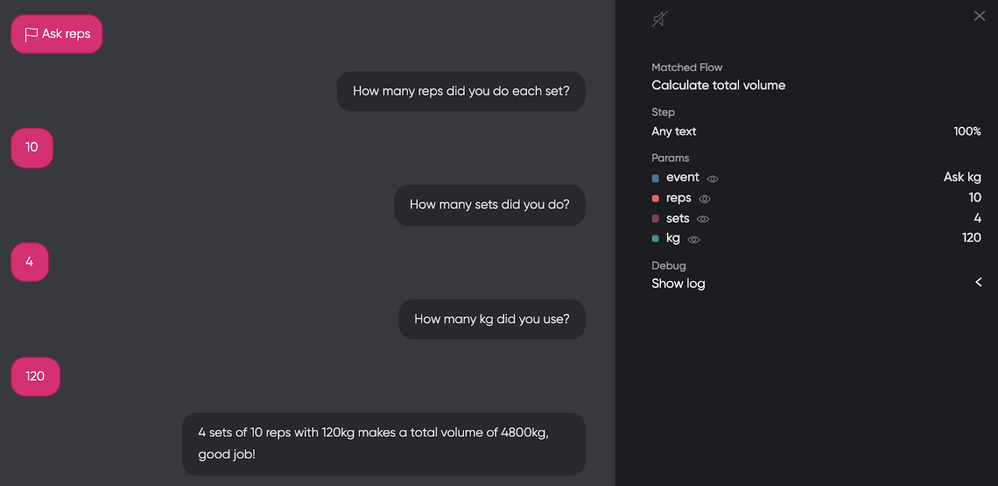Different types of calculations:

You can perform the following basic as well as advanced calculations using the bot:

• Multiplying
• Dividing
• Subtracting

### Different Types of Calculation Questions

The first step is to create an intent that detects when somebody wants the bot to calculate something. Flow can do so by matching the intent with examples as such: Do you understand math? or Can you calculate something for me?

For the above case, you could you can ask the user what the bot should calculate. Sometimes the user already provides specific information about the calculations, like What is 8 divided by 4? or 8/4=?

You can make your calculator bot save that information for future reference. It enhances the user experience since it reduces the effort of the user.

### Creating a calculation Intent

You can create intent to match with the user request for calculations: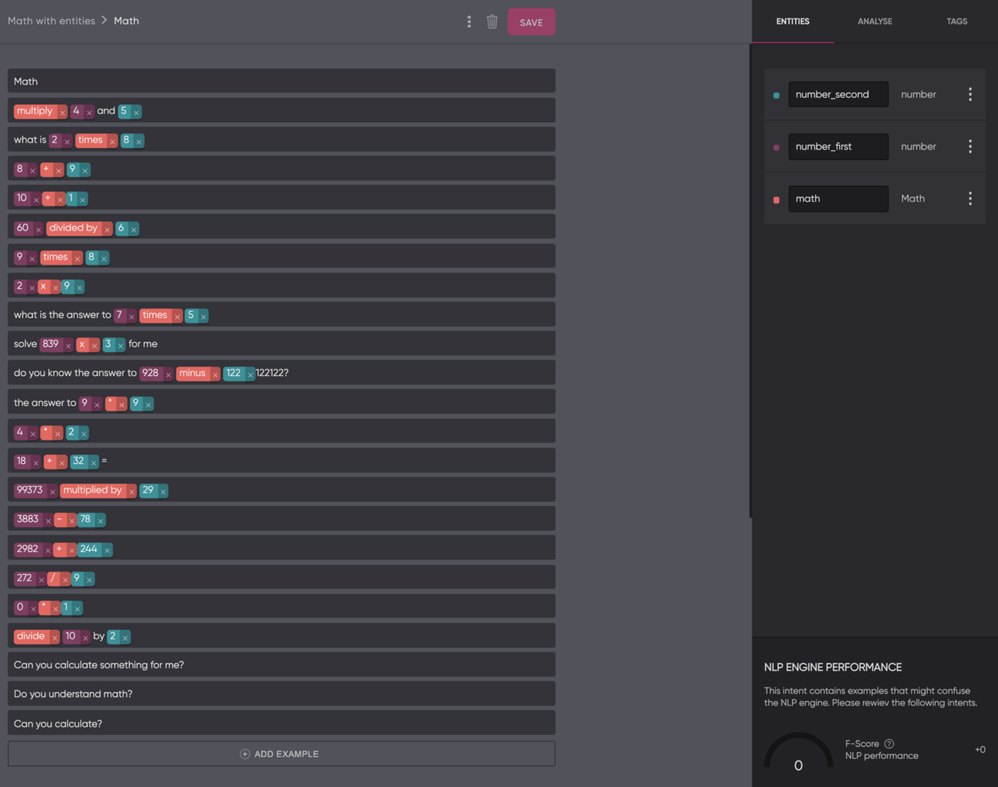In the above example, we have use 3 entities:

• number_first
• number_second
• math

The first and second numbers in the intent are separately captured because for dividing and subtracting, you need to know which number should be divided or subtracted from the other number. Both number entities use the default system entity for numbers.

## The Math Entity

The third entity (math) is a custom entity. It contains the 4 types of math we already discussed briefly, along with some synonyms for them: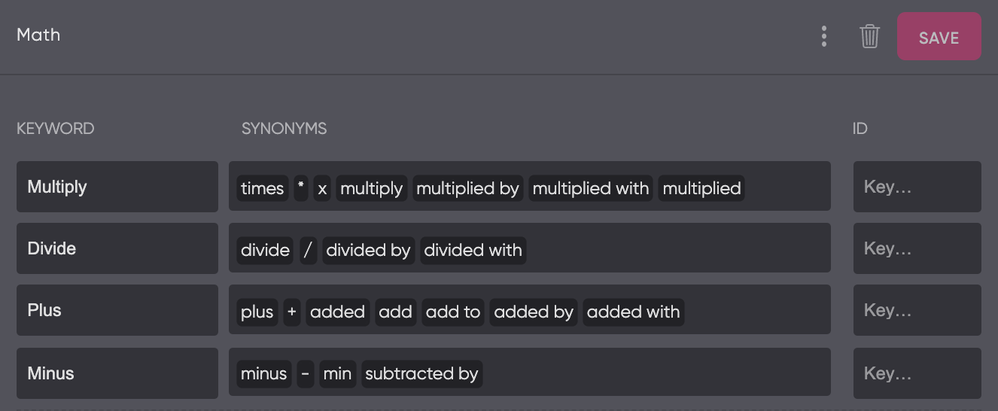## Requiring all Entities

Now we have created the entity and the intent, let's design the flow.

All 3 entities need to have a value to enable the bot to solve the calculation, so the first step is to set all of them to be required: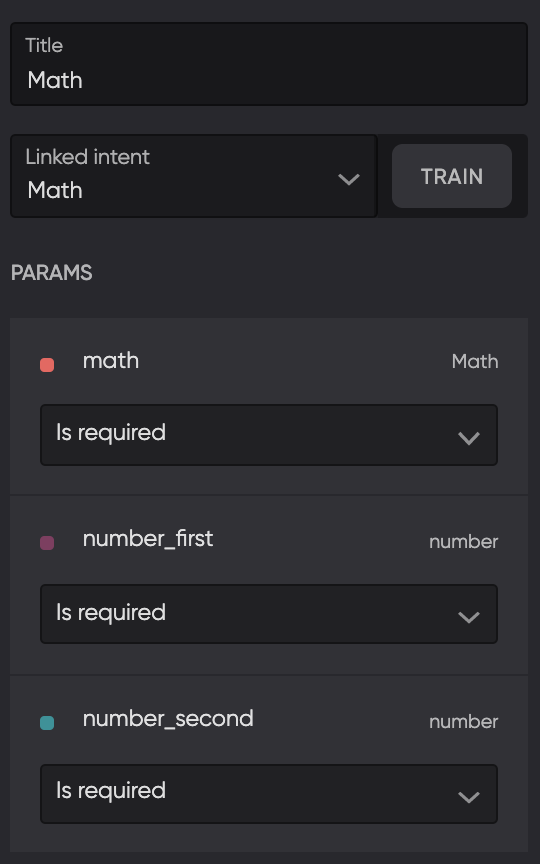This will add sections under the intent for when one of the entities isn’t known after matching the intent. Click on all of them and add a Text Reply that instructs to give it a value.

### Mathematical Conditions

Since we have 4 types of calculation, we need to add 4 conditions that check which type the condition bot should use. The image below shows how to check with a condition if the math type is Minus. Do the same for Multiply, Divide, and Plus.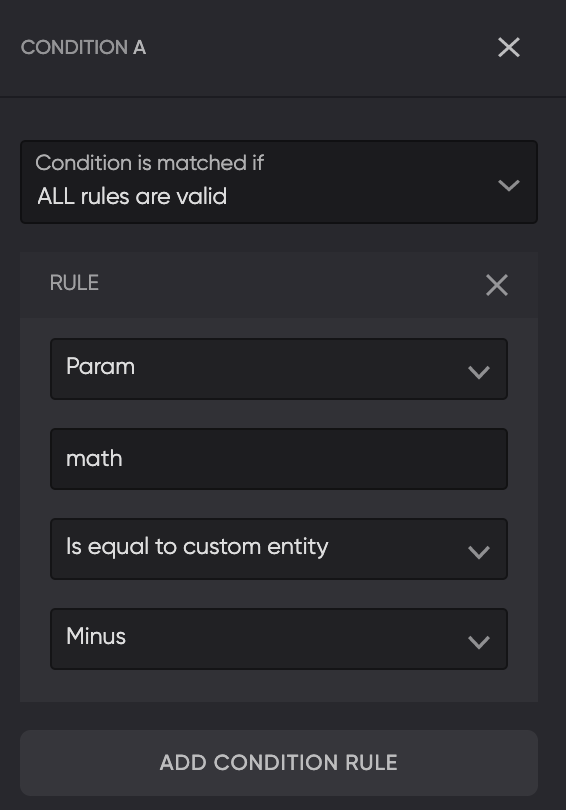Also, add a 5th Else condition without any rules, so we can give an error message when the bot isn’t able to match with any of the conditions due to some unforeseen problem we missed.

### String Templates

To calculate the value of the entity number_first minus the value of number_second, use the following String Template:

{{number_first.first.match | minus: number_second.first.match}}

If you change “minus” to the other math types, you get the following flow: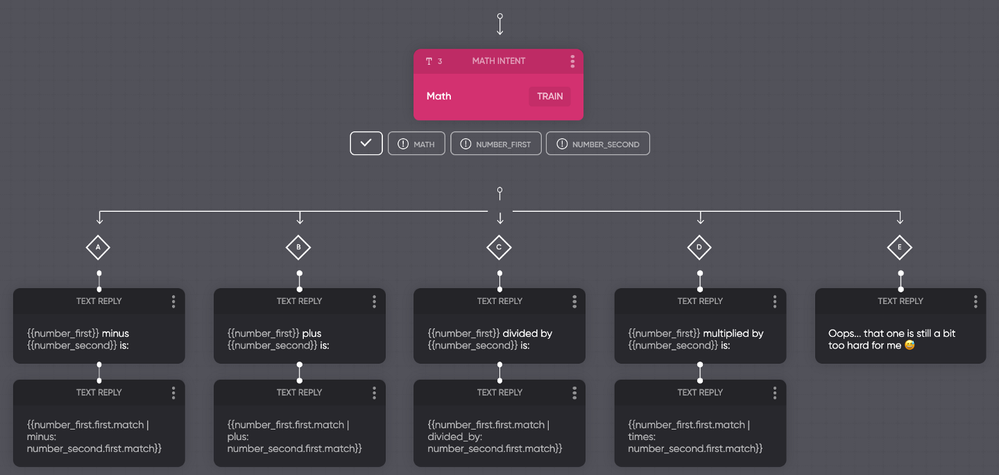### Resetting parameters

When we test our flow twice, you can see the second time it doesn’t work correctly yet. The parameter number_first still contains the value from our first test.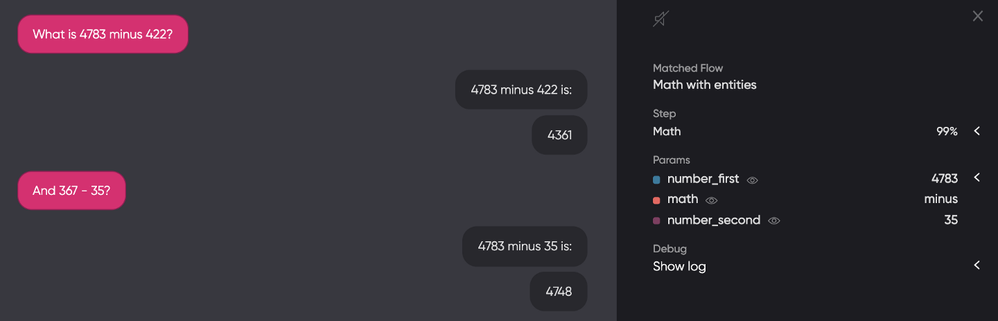That is because we need to reset parameters before giving them new values. Reset the values after each response, as shown in the image below.As you can see, the problem has now been solved!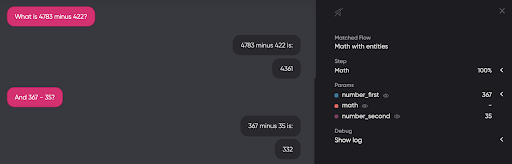And that’s how you teach math to your bot!

There are way more possibilities with String Templates, so have a look at our documentation if you want to learn more.